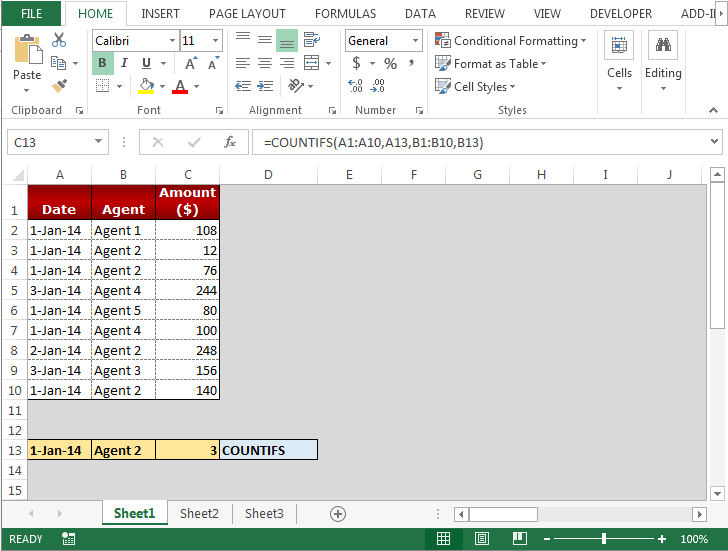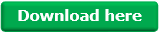# AVERAGEIFS, SUMIFS and COUNTIFS Functions in Microsoft Excel

In this article, we will learn how to apply “Averageifs”, “Sumifs” and “Countifs” in Microsoft Excel 2013.

AVERAGEIFS: Finds average (arithmetic mean) for the cells specified by a given set of conditions or criteria.

Syntax: =AVERAGEIFS(average_range,criteria_range,criteria,...)

average_range: The range of cells for that you want to derive an average.

criteria_range: The range of cells from which you want criteria to evaluate.

Criteria: The criteria define which cells to average.

Let’s take an example to understand how and where we can use the Averageifs Excel function.

We have data in range A1:C10. Column A contains Date, Column B contains Agent and Column C contains Amount. We want to return the average to the amount for an Agent and date wise.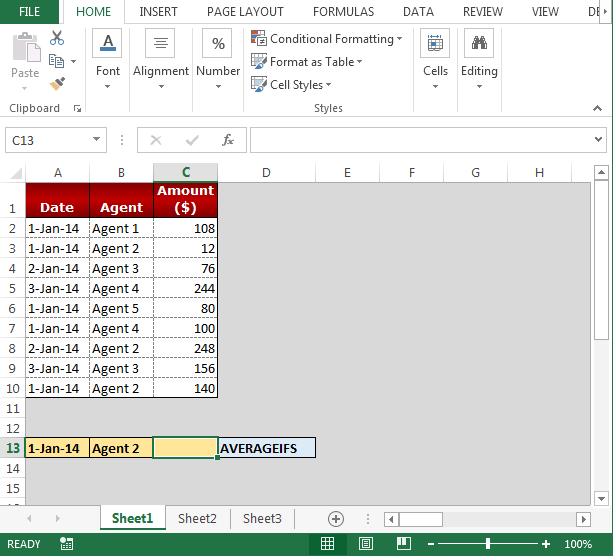Follow below given steps to return the average for two criteria:-

• Select the cell C13, and write the formula.
• =AVERAGEIFS(C1:C10,A1:A10,A13,B1:B10,B13)
• Press Enter on your keyboard.
• You will get the average amount according to an Agent and date wise.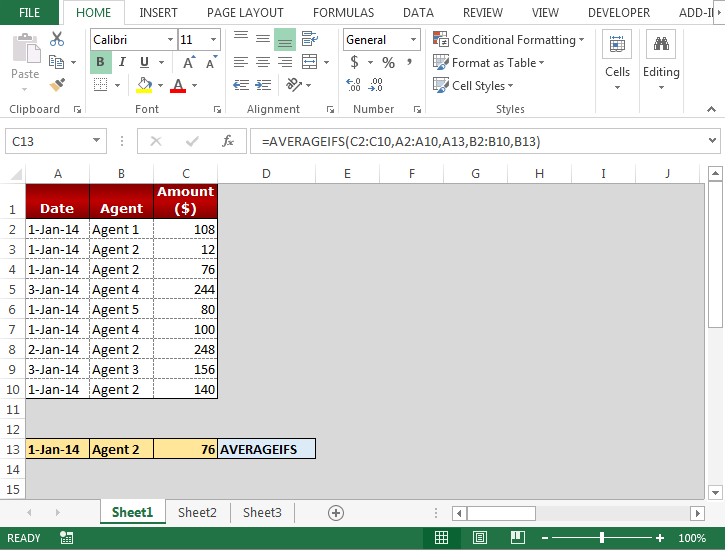SUMIFS function is used for adding the cells specified by a given set of condition or criteria.

The syntax of SUMIFS formula:-  =SUMIFS(sum_range,criteria_range1,criteria1,…)

How to use SUMIFS formula in Excel?

We want to return the total to the amount for an Agent and date wise.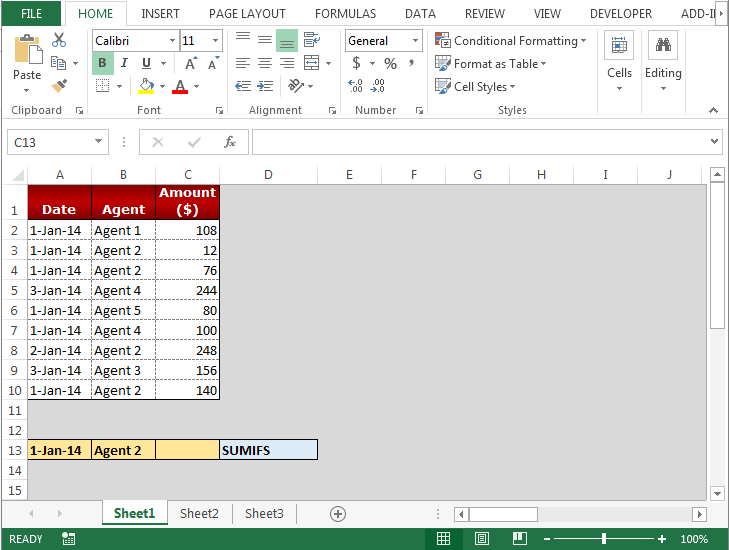Follow below given steps to return the total according to two criteria:-

• Select the cell C13, and write the formula.
• =SUMIFS(C1:C10,A1:A10,A13,B1:B10,B13)
• Press Enter on your keyboard.
• You will get the total amount according to an Agent and date wise.COUNTIFS function is used to count the entries in multiple range based on multiple criteria.

Syntax of “COUNTIFS” function:

=COUNTIFS (criteria_range1, criteria1, [criteria_range2, criteria2]…)

How to use COUNTIFS function in Excel?

We want to return the total of the amount for an Agent and date wise.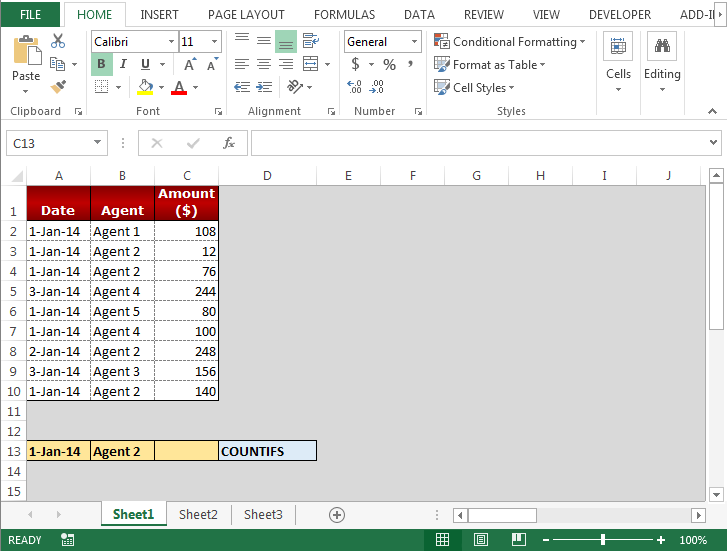Follow below given steps to return the total according to two criteria:-

• Select the cell C13, and write the formula.
• =COUNTIFS(A1:A10,A13,B1:B10,B13)
• Press Enter on your keyboard.
• You will get the no. of agents according to an Agent and date wise.